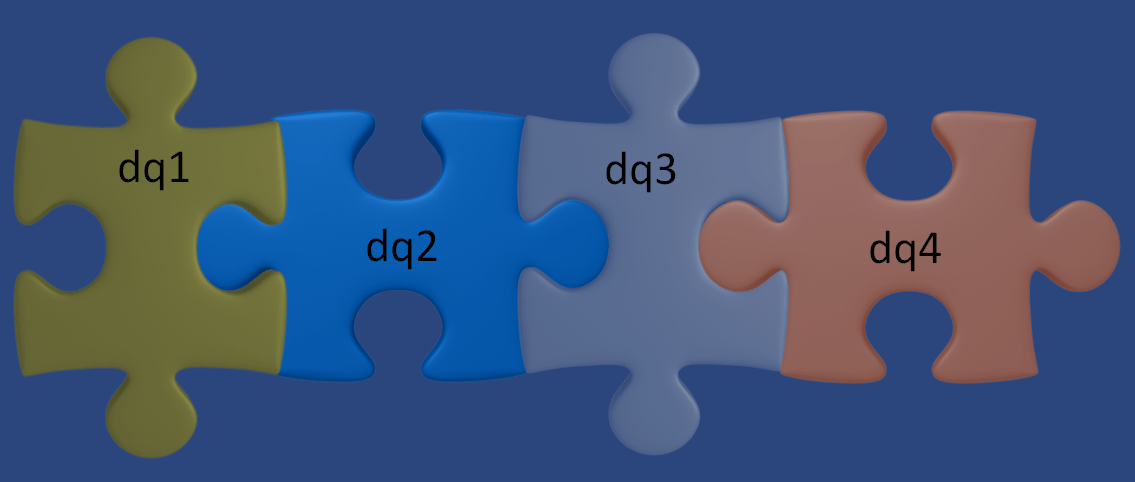#INFOSWMM

How is the St Venant Equation Solved for in the Dynamic Wave Solution of SWMM 5?

Subject:   How is the St Venant Equation Solved for in the Dynamic Wave Solution of SWMM 5?

An explanation of the four StVenant Terms in SWMM 5 and how they change for Gravity Mains and Force Mains. The HGL is the water surface elevation in the upstream and downstream nodes of the link. The HGL for a full link goes from the pipe crown elevation up to the rim elevation of the node + the surcharge depth of the node.  The four terms are:

dq2 = Time Step * Awtd * (HGL) / Link Length

Qnew = (Qold – dq2 + dq3 + dq4) / ( 1 + dq1)

when the force main is full dq3 and dq4 are zero and

Qnew = (Qold – dq2) / ( 1 + dq1)

The dq4 term in dynamic.c uses the area upstream (a1) and area downstream (a2), the midpoint velocity, the sigma factor (a function of the link Froude number), the link length and the time step or

dq4 = Time Step * Velocity * Velocity * (a2 – a1) / Link Length * Sigma

the dq3 term in dynamic.c uses the current midpoint area (a function of the midpoint depth), the sigma factor and the midpoint velocity

dq3 = 2 * Velocity * ( Amid(current iteration) – Amid (last time step) * Sigma

dq1 = Time Step * RoughFactor / Rwtd^1.333 * |Velocity|

The weighted area (Awtd) is used in the dq2 term of the StVenant equation:

The four terms change at each iteration and time step to determine the new flow (Figure 1) based on the two equations:

Denom = 1 + dq1 + dq5

Q = [Qold – dq2 + dq3 + dq4] / Denom

If you look at a table of the values you will see that the terms add up to zero when the flow is constant and to delta Q or the change in Q when the flow is NOT constant (Figure 2).Figure 1.  The four terms define the new flow at each iteration in the dynamic wave solution of SWMM5Figure 2.   The magnitude of the four terms determine the flow at the new iteration and ultimately the new Time Step.  If the flow is constant then the value of the term is constant.